# Modular group algebra

Jump to: navigation, search

Letbe a field anda group. The group algebra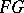is called modular if the characteristic ofis prime, say, andcontains an element of order; otherwiseis said to be non-modular.

Practically every result about group algebras over a field of characteristic zero has an appropriate analogue for any non-modular group algebra. Absence of finite skew-fields makes it possible to state certain prime-characteristic analogues in a stronger form. For example, ifis a finite group, then a non-modular group algebrain characteristic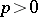is a sum of matrix algebras over fields (rather than over skew-fields, as in the case of characteristic zero; cf. also Matrix algebra). The theory of modular group algebras is of a much higher level of complexity than that of the non-modular group algebras. For finite, the topic essentially belongs to the theory of modular representations of finite groups (cf. Finite group, representation of a), and includes such rich pieces as the theory of blocks (cf. Block), Brauer correspondences, the theory of projective and relatively projective modules (cf. Projective module), etc. The radical theory of modular group algebras forfinite is not very well developed (1996). E.g., there are only fragmentary results about the dimension and the nilpotency index of the radical.

However, ifis infinite, the most studied case is precisely the theory of radicals, mainly the Jacobson radical. The results obtained centre around two conjectures. The first conjecture, proved for locally solvable groups (cf. Solvable group), states that, the Jacobson radical of, coincides with the so-called-radical, whereis defined as the set of all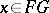such that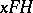is nilpotent (cf. Nilpotent group) for every finitely generated subgroup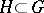. Setand letbe the subgroup of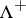generated by the-elements. Thenis locally finite (i.e., every finite set is contained in a finite subgroup) and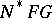is generated by the Jacobson radical of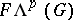. This reduces the problem to locally finite groups.

The second conjecture concerns locally finite groups and has recently been settled affirmatively by D.S. Passman [a2]. To state it, let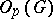be the maximal normal-subgroup of the locally finite groupand let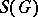be the subgroup generated by all finite subgroups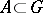such thatis generated by-elements andis a subnormal subgroup in every finite subgroup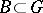with. Letbe the pre-image ofin. Then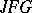is generated (as an ideal) by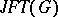, andis easily described in terms of radicals of the group rings of finite groups. Thus, the problem is in fact reduced to the case of finite groups, which is usually regarded as a satisfactory solution for a problem in the theory of infinite groups.

For a locally finite, the two-sided idealsofsuch that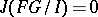can be described in terms of the representations of finite subgroups of, [a3]. This also provides an efficient machinery for studying the lattice of two-sided ideals of.